# Acoustics of Concert Halls and RoomsPage 1

#### WATCH ALL SLIDES

Slide 1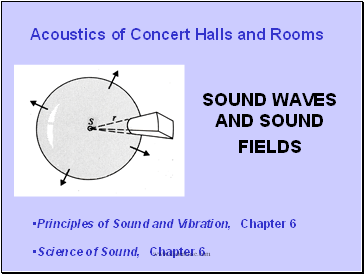## Acoustics of Concert Halls and Rooms

SOUND WAVES AND SOUND FIELDS

Principles of Sound and Vibration, Chapter 6

Science of Sound, Chapter 6

Slide 2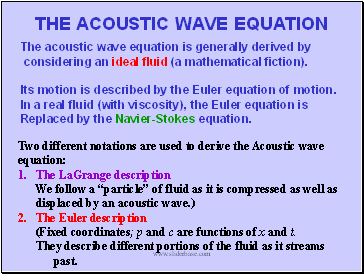## The acoustic wave equation

The acoustic wave equation is generally derived by

considering an ideal fluid (a mathematical fiction).

Its motion is described by the Euler equation of motion.

In a real fluid (with viscosity), the Euler equation is

Replaced by the Navier-Stokes equation.

Two different notations are used to derive the Acoustic wave

equation:

The LaGrange description

We follow a “particle” of fluid as it is compressed as well as displaced by an acoustic wave.)

The Euler description

(Fixed coordinates; p and c are functions of x and t.

They describe different portions of the fluid as it streams past.

Slide 3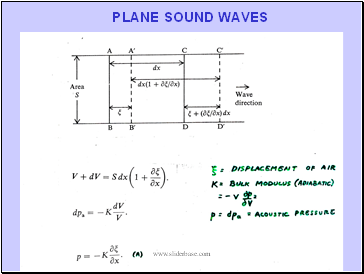PLANE SOUND WAVES

Slide 4Plane Sound Waves

Slide 5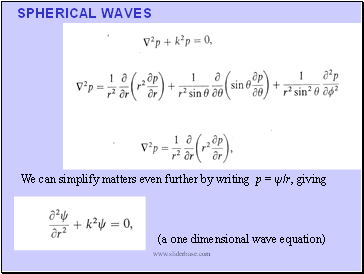SPHERICAL WAVES

We can simplify matters even further by writing p = ψ/r, giving

(a one dimensional wave equation)

Slide 6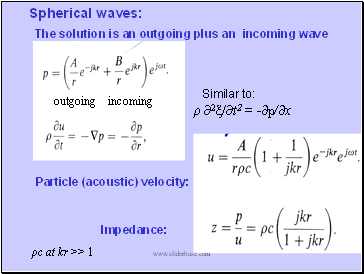Spherical waves:

Particle (acoustic) velocity:

Impedance:

The solution is an outgoing plus an incoming wave

ρc at kr >> 1

Similar to:

ρ ∂2ξ/∂t2 = -∂p/∂x

outgoing incoming

Slide 7SOUND PRESSURE, POWER AND LOUDNESS

Decibels

Decibel difference between two power levels:

ΔL = L2 – L1 = 10 log W2/W1

Sound Power Level: Lw = 10 log W/W0 W0 = 10-12 W

(or PWL)

Sound Intensity Level: LI = 10 log I/I0 I0 = 10-12 W/m2

(or SIL)

Slide 8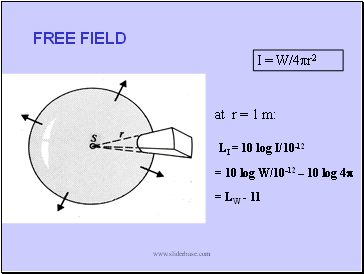FREE FIELD

I = W/4πr2

at r = 1 m:

LI = 10 log I/10-12

= 10 log W/10-12 – 10 log 4p

= LW - 11

Slide 9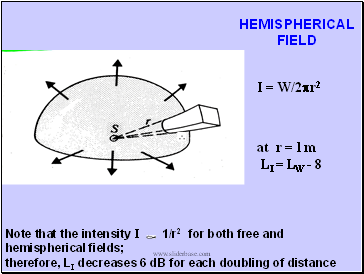HEMISPHERICAL FIELD

I = W/2pr2

at r = l m

LI = LW - 8

Note that the intensity I 1/r2 for both free and hemispherical fields;

therefore, LI decreases 6 dB for each doubling of distance

Slide 10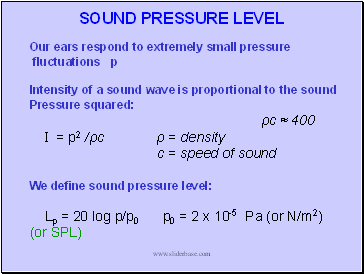## Sound pressure level

Our ears respond to extremely small pressure

fluctuations p

Intensity of a sound wave is proportional to the sound

Go to page:
1  2